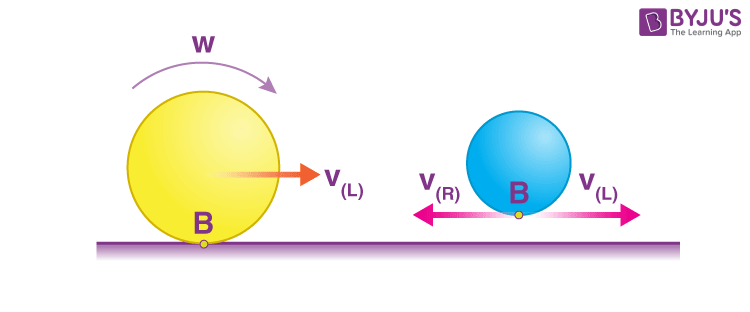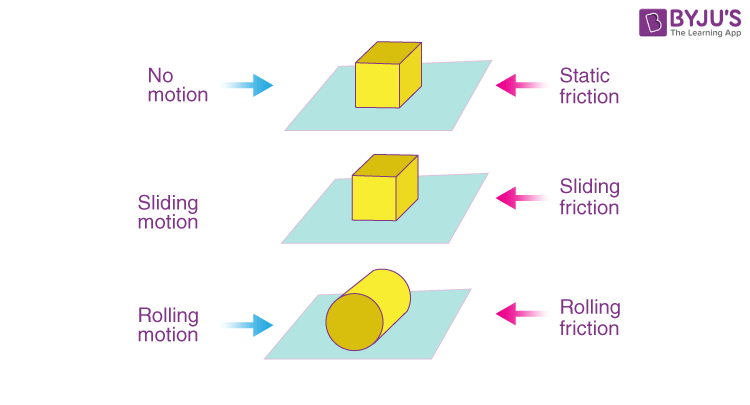# Types of Friction

Friction is the force that opposes the motion of a solid object over another. There are mainly four types of friction: static friction, sliding friction, rolling friction, and fluid friction. Friction and normal force are directly proportional to the contacting surfaces, and it doesn’t depend on the hardness of the contacting surface. With the increase in relative speeds, the sliding friction reduces, whereas fluid friction increases with the increase in the relative speed. Also, fluid friction is dependent on the fluid’s viscosity.

In sliding motion, each point on the body has only translational or linear motion. But in the case of rolling motion, different points have a combination of linear and rotational motion. Look at the following figure:Figure displaying rolling motion

If we consider only the rotational motion, then point B tends to move backwards suppose with a velocity VR. Similarly, we consider only linear motion then point B moves with a velocity VL in the forward direction. Now the resultant motion of the point B will be a superposition of both the above velocities. Depending upon the values of VR and VL the rolling friction can act in the following directions:

If VR > VL then the point B tends to move backwards so as we know, friction opposes relative motion between the surfaces in contact; hence it will act in the forward direction.

Similarly, if VR < VL then the point B tends to move forward and hence the friction acts in the backward direction.

Also, if VR = VL although the ball moves in the forward direction, the frictional force acting on the ball will be zero. This is unlike sliding friction where the friction force will definitely act if the body is moving.Types Of friction

## Types Of Friction

Following are the friction types which depend on the types of motion:

1. Static Friction
2. Sliding Friction
3. Rolling Friction
4. Fluid Friction

## Static Friction

Static friction is defined as the frictional force that acts between the surfaces when they are at rest with respect to each other.

The magnitude of the static force is equal in the opposite direction when a small amount of force is applied. When the force increases, at some point maximum static friction is reached.

### Static Friction Examples

Following are the examples of static friction:

• Skiing against the snow
• Creating heat by rubbing both the hands together
• Table lamp resting on the table

### Coefficient Of Static Friction

The coefficient of static friction is denoted as µs. The maximum force of static friction is given as the product of the coefficient of static friction and normal force and force of static friction is less than or equal to the product of the coefficient of static friction and normal force. It is given as:

 Fs max = µs η and Fs ≤ µs η

Where,

• Fis the force of static friction
• µs is the coefficient of static friction
• η is the normal force
• Fmax is the maximum force of static friction

## While solving the problem given in the video, you will understand how friction changes from static to kinetic## Sliding Friction

### What Is Sliding Friction?

Sliding friction is defined as the resistance that is created between any two objects when they are sliding against each other.

### Examples Of Sliding Friction

Following are the examples of sliding friction:

• Sliding of the block across the floor
• Two cards sliding against each other in a deck

### Coefficient Of Sliding Friction

The coefficient of sliding friction is denoted as µs. The force of sliding friction is defined as the product of the coefficient of sliding friction and the normal force. It is given as:

 Fs = µs. Fn

## Rolling Friction

### What Is Rolling Friction?

Rolling friction is defined as the force which resists the motion of a ball or wheel and is the weakest types of friction.

### Examples Of Rolling Friction

Following are the examples of rolling friction:

• Rolling of the log on the ground
• Wheels of the moving vehicles

### Coefficient Of Rolling Friction

The coefficient of rolling friction is denoted as µr. The force of rolling friction is defined as the product of the coefficient of rolling friction and the normal force. It is given as:

 Fs = µr. Fn

## Fluid Friction

### What Is Fluid Friction?

Fluid friction is defined as the friction that exists between the layers of the fluid when they are moving relative to each other.

### Examples Of Fluid Friction

Following are the examples of fluid friction:

• The flow of ink in pens
• Swimming

## Understand the variable and self-adjusting nature of the static friction with the help of the animation provided in the video below## Frequently Asked Questions – FAQs

Q1

### What is static friction?

Static friction is defined as the frictional force that acts between the surfaces when they are at rest with respect to each other.
Q2

### What is sliding friction?

Sliding friction is defined as the resistance that is created between any two objects when they are sliding against each other.
Q3

### Define rolling friction.

Rolling friction is defined as the force which resists the motion of a ball or wheel and is the weakest type of friction.
Q4

### What is fluid friction?

Fluid friction is defined as the friction that exists between the layers of the fluid when they are moving relative to each other.
Q5

### Give two examples of sliding friction.

Sliding of the block across the floor and two cards sliding against each other in a deck.